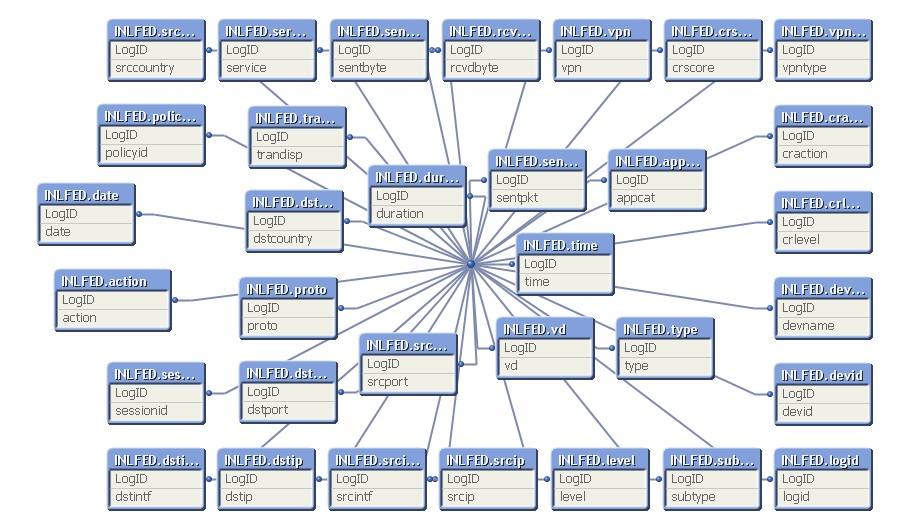# QlikView Scripting

Discussion Board for collaboration on QlikView Scripting.

New Contributor III

## Indexing Script

Hi Guys

Can any of you help me to index this code in fields and fieldvalues.

This is one string from a log file.

Need a backend script to decode into fields and fieldnames for qlikview

Jul 20 20:06:27 firewall.ip date=2016-07-20 time=21:15:56 devname=FIREWALL.HOSTNAME devid=FIREWALL.SERIAL.NUMBER logid=0000000013 type=traffic subtype=forward level=notice vd=root srcip=SOURCE.IP srcport=1031 srcintf="SOURCE.INTERFACE" dstip=DESTINATION.IP dstport=514 dstintf="wan1" sessionid=47632605 proto=17 action=deny policyid=0 dstcountry="South Africa" srccountry="Reserved" trandisp=noop service="SYSLOG" duration=0 sentbyte=0 rcvdbyte=0 sentpkt=0 vpn="VPN.TUNNEL.NAME" vpntype=ipsec-static appcat="unscanned" crscore=30 craction=131072 crlevel=high

Thank you

Kind regards

Tags (1)
1 Solution

Accepted Solutions
Honored Contributor

## Re: Indexing Script

Hi Chris,

Try this;

Generic

LogID,

SubField(Log,'=',1) as Attribute,

SubField(Log,'=',2) as Value;

* Where Index(Log,'=');

LogID,

SubField(Log,' ') as Log;

LogID,Log

1,'Jul 20 20:06:27 firewall.ip date=2016-07-20 time=21:15:56 devname=FIREWALL.HOSTNAME devid=FIREWALL.SERIAL.NUMBER logid=0000000013 type=traffic subtype=forward level=notice vd=root srcip=SOURCE.IP srcport=1031 srcintf="SOURCE.INTERFACE" dstip=DESTINATION.IP dstport=514 dstintf="wan1" sessionid=47632605 proto=17 action=deny policyid=0 dstcountry="South Africa" srccountry="Reserved" trandisp=noop service="SYSLOG" duration=0 sentbyte=0 rcvdbyte=0 sentpkt=0 vpn="VPN.TUNNEL.NAME" vpntype=ipsec-static appcat="unscanned" crscore=30 craction=131072 crlevel=high'

];

You get a really funky data model;It works but if you want to tidy things up into one table then this further script will do so:

let vTabName = TableName(0);

RENAME Table \$(vTabName) to LogTable;

Let vCnt = NoOfTables();

trace \$(vCnt);

For i = 1 to \$(vCnt)-1

let vOldTabName = TableName(\$(i));

let vNewTabName = Text('Table' &\$(i));

RENAME Table \$(vOldTabName) to \$(vNewTabName);

Next i;

For i = 1 to \$(vCnt)-1

Let vJoinTable =Text('Table' & \$(i));

Left Join(LogTable)

DROP Table \$(vJoinTable);

next i;

now the model looks a bit more conventionalCheers

Andrew

4 Replies
Honored Contributor II

## Re: Indexing Script

I was too lazy to work out the whole script for you.

I include enough fields so you could see:

fixed width fields (date and time)

fields terminated by a space (devname and devid)

fields with data in double quotes that might contain a space (dstcountry)

Honored Contributor

## Re: Indexing Script

Hi Chris,

Try this;

Generic

LogID,

SubField(Log,'=',1) as Attribute,

SubField(Log,'=',2) as Value;

* Where Index(Log,'=');

LogID,

SubField(Log,' ') as Log;

LogID,Log

1,'Jul 20 20:06:27 firewall.ip date=2016-07-20 time=21:15:56 devname=FIREWALL.HOSTNAME devid=FIREWALL.SERIAL.NUMBER logid=0000000013 type=traffic subtype=forward level=notice vd=root srcip=SOURCE.IP srcport=1031 srcintf="SOURCE.INTERFACE" dstip=DESTINATION.IP dstport=514 dstintf="wan1" sessionid=47632605 proto=17 action=deny policyid=0 dstcountry="South Africa" srccountry="Reserved" trandisp=noop service="SYSLOG" duration=0 sentbyte=0 rcvdbyte=0 sentpkt=0 vpn="VPN.TUNNEL.NAME" vpntype=ipsec-static appcat="unscanned" crscore=30 craction=131072 crlevel=high'

];

You get a really funky data model;It works but if you want to tidy things up into one table then this further script will do so:

let vTabName = TableName(0);

RENAME Table \$(vTabName) to LogTable;

Let vCnt = NoOfTables();

trace \$(vCnt);

For i = 1 to \$(vCnt)-1

let vOldTabName = TableName(\$(i));

let vNewTabName = Text('Table' &\$(i));

RENAME Table \$(vOldTabName) to \$(vNewTabName);

Next i;

For i = 1 to \$(vCnt)-1

Let vJoinTable =Text('Table' & \$(i));

Left Join(LogTable)

DROP Table \$(vJoinTable);

next i;

now the model looks a bit more conventionalCheers

Andrew

New Contributor III

## Re: Indexing Script

Thanks Andrew,

Nice and simple answerHonored Contributor II

## Re: Indexing Script

This answer is nice and simple, but doesn't get data where there is a space between double quotes (South Africa)